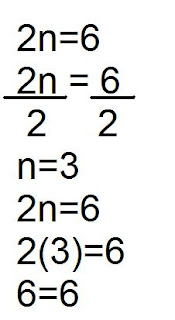### The Great Big Book Of Algebra

Thursday, December 4, 2008
Chapter One

All together, combined, plus,
Gaining integer

Return to list of postsHaiku - Subtracting Integers

Subtract

Subtract property,
Subtract, minus integers,

Free Verse - Ron's Rule

Ron's Rule

If you want to pass
a math test
just follow Ron's easy quest
he made this rule
it sound pretty cool
if you want to hear it
give him some time
that homie still making it fine
last time I checked
it went little something like this
multiplying odd numbers of
negative integers
makes a negative product

Free verse - Partitive Division

Partitive

If you have division
integer questions
and it has a negative
on the first digit
then it's called
partitive division

Cinquan - Quotative Division
Quoative

positive,negative
writing, thinking, dividing
fun to draw and learn
groups

CHAPTER 2
Combining like terms and the Distributive Property
Script:
Nick "Hey how's it going?"
Alex "I'm doing fine. How about you ?"
Nick "Same here. Well I haven't seen you in the hallways at school in a while."
Alex "Oh that's because I'm been busy helping other students. That's all."
Alex "Hey listen I have a test tomorrow for math."
Alex "Well i kind of need help on it. Will you help me?"
Alex "It's about an algebra question. So, what is n+3-5n+12?"
Nick "Let me think. I think it is 4n+15."
Alex "Oh, well I think the answer is -6n+15."
Nick "Okay. Well, let us see. We first have to circle and regroup."
Alex "So I guess it would be n-5n+3+12."
Nick "Then you must simplify."
Nick "I think the answer is 4n."
Alex "Okay, but I think it's -6n instead of 4n."
Nick "Yes but it says that you have to subtract a number to 5n."
Nick "So the answer for +3+12 is +15!"
Nick "I guess the mistake that you made was that you added n and 5n together instead of subtracting it. Then that's why you got -6n."
Alex "Thank you!"
Nick "You are very welcome."
Nick "Since you asked me that kind of question. Well, I need help too."
Nick "It's a math question too. It's 2 + 4(3n+8)."
Nick "Well I think the an 12n + 10."
Alex "Oh. Let us see what we get with the right steps of how to do this. Okay? So this time we have to first identify the terms. That is 3n and 8.Then you multiply +4 and 3n. You'll get +12n."
Nick "Okay then you have to multiply +4 and +8? Then yeah I get +32"
Nick "Oh okay. Then I guess that's where I did mine wrong. I forgot that you have to multiply the ones in the brackets."
Alex "Well I guess so but let's see if you have anything else wrong."
Alex "Well, at the beginning we should have taken the 2 and bring it down."
Nick "We should have 2+12n+32?"
Alex "Yes! Then like the other question you have to circle and regroup them."
Alex "Then we'll end up with 34+12n."
Nick "Yes I get it now. Thank you for the help."
Alex "You're welcome and thank you for the help also."
Nick "So do you want to go to my house and have snacks?'
Alex "Yes. Okay. I'm starving after doing all this math questions."

Chapter 3 : One step Equation Solving

Here are some additive, subtractive, Multiplicative and divisive eqatuons.I solved this equation by using these 4 steps. First, isolate the variable, second cancel the opposite, third balance and forth verify. So, the first thing I did was I rewrote the equation and added the opposites. Then I canceled the opposite. Then do it on the other side so it would be balanced. So, then you get n=2. But you're not done yet because you have to verify. So I rewrote the question and I replaced the n to 4. So the answer will 5=5.Subtractive:

This is how I solved this equation. First, I rewrote the equation, then I isolated the opposites. After I isolated the oppsites, I went and I canceled the opposites but you have to do it on the other side of the equal sign so it would be balanced. Well you're not done yet because you have to verify. So I rewrote the question then I replaced the n and put 11 because that was my answer earlier. Then the answer in 7=7.Multiplicative:

This is how I solved this equation. So I rewrote the equation like I always do. Then I divided 2n to 2 to isolate the variable and canceled the zero pairs after. I did it on the other side so it would be balanced. So the answer would be n is equal 3. Then you have to varify if my answer was right. So I rewrote the question, then I replaced the n and multiplied 3. Then the answer would be 6=6.

This is how I solved this divisive equation. At first I started to rewrite the question, then I isolated the variable. So I multiplied the 4 to n divided by 4 and I also multiplied 4 and 3. Then I canceled the opposites. So the answer would be n=12. But I wasn't done yet because I haven't varify yet. So I rewrote the question again like I always do. Then I replaced the n and preplac it for 12. So the answer for this equation is 3=3.

Chapter 4: Algetile Video

During class, Mr. Harbeck told us to make a video about algebra equations using algetiles and in writing. We couldn't finish it at school because we kept restarting like 5 times. So I did it for homework this weekend.

Sorry, the last part was cut off because my memory was full but this is what I said "So you have to verify. So, rewrite the question and replace the n and put 8 and your answer will be 4=4."